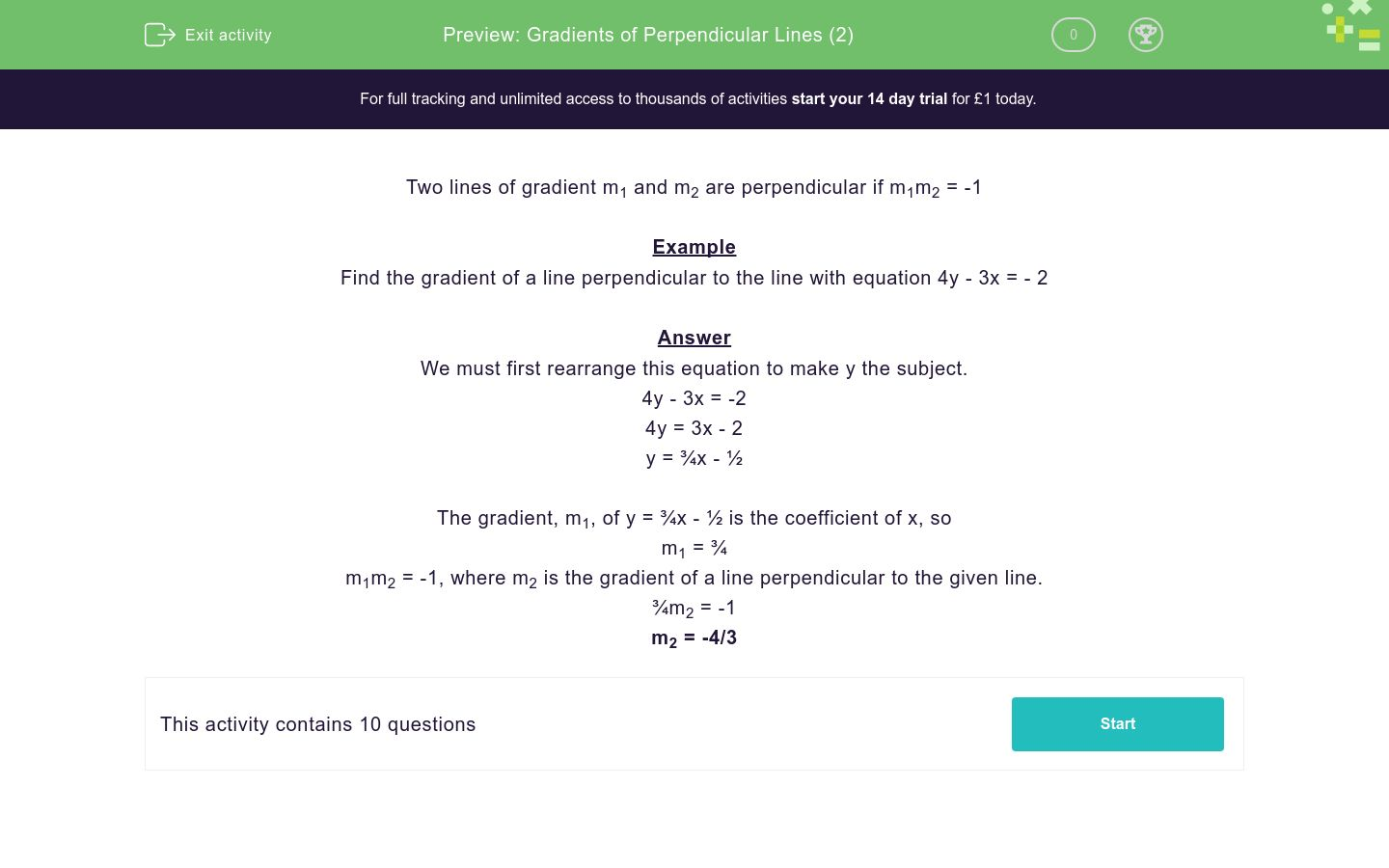# Gradients of Perpendicular Lines (2)

In this worksheet, students state the gradient of a line perpendicular to the given line.Key stage:  KS 4

Curriculum topic:  Algebra

Curriculum subtopic:  Use y = mx + c to Identify Parallel and Perpendicular Lines

Difficulty level:### QUESTION 1 of 10

Two lines of gradient m1 and m2 are perpendicular if m1m2 = -1

Example

Find the gradient of a line perpendicular to the line with equation 4y - 3x = - 2

We must first rearrange this equation to make y the subject.

4y - 3x = -2

4y = 3x - 2

y = ¾x - ½

The gradient, m1, of y = ¾x - ½ is the coefficient of x, so

m1 = ¾

m1m2 = -1, where m2 is the gradient of a line perpendicular to the given line.

¾m2 = -1

m2 = -4/3

Find the gradient of a line perpendicular to the line with equation:

3y = 3x - 4

-1/3

-1

1/3

Find the gradient of a line perpendicular to the line with equation:

4y = -3x - 4

-4/3

-3/4

4/3

Find the gradient of a line perpendicular to the line with equation:

3y = 4 - 3x

-1/3

1

1/3

Find the gradient of a line perpendicular to the line with equation:

4y = 2x + 4

-1/2

-2

1/2

Find the gradient of a line perpendicular to the line with equation:

8y = -2x + 4

4

-2/8

1/4

Find the gradient of a line perpendicular to the line with equation:

4y + x = 7

4

-1

1

Find the gradient of a line perpendicular to the line with equation:

8y - 4x = 40

-1/2

1/2

-2

Find the gradient of a line perpendicular to the line with equation:

11y + 22x = 44

-1/2

-11/44

1/2

Find the gradient of a line perpendicular to the line with equation:

5y - 20x = -12

4

-1/4

1/4

Find the gradient of a line perpendicular to the line with equation:

5x - 10y = 2

-2

1/5

-1/2

• Question 1

Find the gradient of a line perpendicular to the line with equation:

3y = 3x - 4

-1
EDDIE SAYS
y = x - 4/3
1m2 = -1
• Question 2

Find the gradient of a line perpendicular to the line with equation:

4y = -3x - 4

4/3
EDDIE SAYS
y = -¾x - 1
-¾m2 = -1
• Question 3

Find the gradient of a line perpendicular to the line with equation:

3y = 4 - 3x

1
EDDIE SAYS
y = -x + 4/3
-1m2 = -1
• Question 4

Find the gradient of a line perpendicular to the line with equation:

4y = 2x + 4

-2
EDDIE SAYS
y = ½x + 1
½m2 = -1
• Question 5

Find the gradient of a line perpendicular to the line with equation:

8y = -2x + 4

4
EDDIE SAYS
y = -¼x + ½
-¼m2 = -1
• Question 6

Find the gradient of a line perpendicular to the line with equation:

4y + x = 7

4
EDDIE SAYS
y = -¼x + 7/4
-¼m2 = -1
• Question 7

Find the gradient of a line perpendicular to the line with equation:

8y - 4x = 40

-2
EDDIE SAYS
y = ½x + 5
½m2 = -1
• Question 8

Find the gradient of a line perpendicular to the line with equation:

11y + 22x = 44

1/2
EDDIE SAYS
y = -2x + 4
-2m2 = -1
• Question 9

Find the gradient of a line perpendicular to the line with equation:

5y - 20x = -12

-1/4
EDDIE SAYS
y = 4x - 12/5
4m2 = -1
• Question 10

Find the gradient of a line perpendicular to the line with equation:

5x - 10y = 2

-2
EDDIE SAYS
y = ½x - 0.2
½m2 = -1
---- OR ----

Sign up for a £1 trial so you can track and measure your child's progress on this activity.

### What is EdPlace?

We're your National Curriculum aligned online education content provider helping each child succeed in English, maths and science from year 1 to GCSE. With an EdPlace account you’ll be able to track and measure progress, helping each child achieve their best. We build confidence and attainment by personalising each child’s learning at a level that suits them.

Start your £1 trial